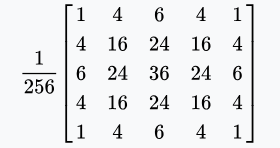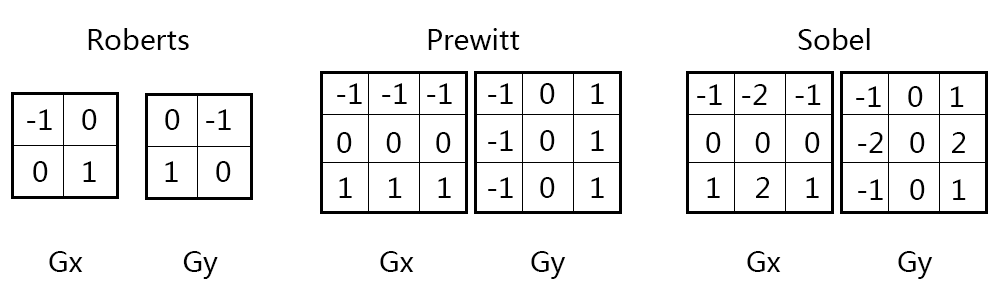## 数据精度的选择

Shader中的绝大多数计算都是通过浮点数来完成的。其中包括几种不同的类型：floathalffixed（矢量和矩阵也有对应的类型如half3float4x4）。这些类型的精度、性能和能耗都有差异：

• 高精度：float

最高精度的浮点数，通常是32位。常用在世界空间的位置坐标、纹理坐标或涉及到复杂函数的标量计算中（如三角函数、幂运算、指数运算）。

• 中等精度：half

中等精度的浮点数，通常是16位（范围是-60000到+60000，精度大约在小数点后三位）。通常用于短的矢量、方向或物体空间的坐标及HDR的颜色。

• 低精度：fixed

最低精度的浮点数，通常11位。范围是-2.0到+2.0，精度为1/256。常用在普通的颜色（保存在普通纹理）及相关计算中。

• 整数类型

整数类型int常用于循环计数或数组索引。这种使用方式通常在跨平台时可以正常工作。但取决于平台，有的GPU不支持int类型。如Direct3D 9和OpenGL ES 2.0只能运算浮点数，因此整数表达式通常是使用复杂的浮点数学指令模拟出来。而Direct3D 11、OpenGL ES 3、Metal和其它的现代平台会提供对整数的支持，可以正常使用位移（bit shifts）和位掩码（bit masking）计算。

• 对于世界位置坐标和纹理坐标，使用float精度
• 其它的一切（矢量、HDR颜色等）可先使用half，如果需要的话再增加精度
• 对于纹理数据的一些简单操作，使用fixed精度

• 所有的现代桌面系统GPU总是会使用float精度来计算，所以使用float/half/fixed实际最终都会是一样的。这样就会使测试变得很困难，因为无法确认half/fixed是否可以真的满足要求，因此必须要在目标设备上进行测试以获得准确的结果。
• 移动平台GPU实际上会使用half精度。这样通常更快，计算时能耗更少。
• fixed精度通常用在旧的移动GPU上。大多数现代GPU（可运行OpenGL ES 3或Metal）内部会同等对待fixedhalf

## 切线空间到世界空间的转换

$$\begin{bmatrix} Tangent_{x} & Bitangent_{x} & Normal_{x} \\ Tangent_{y} & Bitangent_{y} & Normal_{y} \\ Tangent_{z} & Bitangent_{z} & Normal_{z} \end{bmatrix} * \begin{bmatrix} X_T \\ Y_T \\ Z_T \end{bmatrix} = \begin{bmatrix} X_W \\ Y_W \\ Z_W \end{bmatrix}$$

v2f中定义了三个half3用于存储TBN矩阵的三行，在frag中与切线空间的法线tnormal相乘得出世界空间的法线worldNormal

## 卷积和卷积核

### 高斯模糊

$$f(x,y)=\frac{1}{{2\pi}\sigma^2}e^{-\frac{x^2+y^2}{2\sigma^2}}$$x1的卷积核）。需要使用两个pass来绘制，并且需要额外存储第经过一个pass处理之后的纹理。但是复杂度从H*W*5*5变为HW\(5+5)。

### 边缘检测$$G = \sqrt{G_x^2 + G_y^2}$$

$$G = \vert{G_x}\vert + \vert{G_y}\vert$$

## REFERENCE

https://docs.unity3d.com/Manual/SL-DataTypesAndPrecision.html

https://docs.unity3d.com/Manual/SL-ShaderPerformance.html

https://docs.unity3d.com/Manual/SL-VertexFragmentShaderExamples.html

http://www.songho.ca/dsp/convolution/convolution.html

《Unity Shader入门精要》